## 9.2.1 Modeling Nature

For the first time in this book, uncertainty will be directly modeled. There are two DMs:

1. [] Robot: This is the name given to the primary DM throughout the book. So far, there has been only one DM. Now that there are two, the name is more important because it will be used to distinguish the DMs from each other.
2. [] Nature: This DM is a mysterious force that is unpredictable to the robot. It has its own set of actions, and it can choose them in a way that interferes with the achievements of the robot. Nature can be considered as a synthetic DM that is constructed for the purposes of modeling uncertainty in the decision-making or planning process.

Imagine that the robot and nature each make a decision. Each has a set of actions to choose from. Suppose that the cost depends on which actions are chosen by each. The cost still represents the effect of the outcome on the robot; however, the robot must now take into account the influence of nature on the cost. Since nature is unpredictable, the robot must formulate a model of its behavior. Assume that the robot has a set,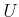, of actions, as before. It is now assumed that nature also has a set of actions. This is referred to as the nature action space and is denoted by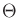. A nature action is denoted as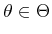. It now seems appropriate to callthe robot action space; however, for convenience, it will often be referred to as the action space, in which the robot is implied.

This leads to the following formulation, which extends Formulation 9.1.

Formulation 9..3 (A Game Against Nature)
1. A nonempty setcalled the (robot) action space. Each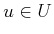is referred to as an action.
2. A nonempty setcalled the nature action space. Eachis referred to as a nature action.
3. A function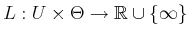, called the cost function.

The cost function,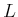, now depends onand. Ifandare finite, then it is convenient to specifyas a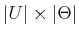matrix called the cost matrix.

Example 9..8 (A Simple Game Against Nature)   Suppose thatandeach contain three actions. This results in nine possible outcomes, which can be specified by the following cost matrix: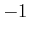0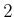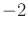The robot action,, selects a row, and the nature action,, selects a column. The resulting cost,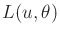, is given by the corresponding matrix entry.In Formulation 9.3, it appears that both DMs act at the same time; nature does not know the robot action before deciding. In many contexts, nature may know the robot action. In this case, a different nature action space can be defined for every. This generalizes Formulation 9.3 to obtain:

Formulation 9..4 (Nature Knows the Robot Action)
1. A nonempty setcalled the action space. Eachis referred to as an action.
2. For each, a nonempty set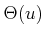called the nature action space.
3. A function, called the cost function.

If the robot chooses an action, then nature chooses from.

Steven M LaValle 2020-08-14